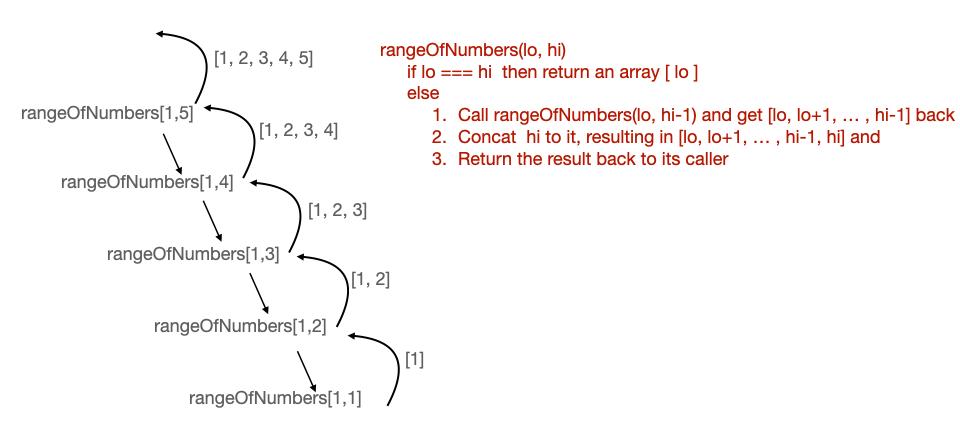# Can't Understand how Use Recursion to Create a Range of Numbers

Hi, please, could somebody explain to me this piece of code? i can’t get how it works
Thanks

``````
function rangeOfNumbers(startNum, endNum) {
return startNum === endNum
? [startNum]
: rangeOfNumbers(startNum, endNum - 1).concat(endNum);
}
``````
``````  **Your browser information:**
``````

User Agent is: `Mozilla/5.0 (Windows NT 10.0; Win64; x64) AppleWebKit/537.36 (KHTML, like Gecko) Chrome/90.0.4430.93 Safari/537.36`.

Challenge: Use Recursion to Create a Range of Numbers

Hi, let’s break this down bit by bit.
We first start and see the ternary operation in the form of
`(boolean expression) ? (code to execute if true) : (code to execute if false);`

In this case, if the given inputs `startNum` and `endNum` are equal, then we return an array with one item: `startNum`
if the two inputs are not equal, then we call the function `rangeOfNumbers` (which is itself) again.

So in terms of if/else, the code can be rewritten as:

``````function rangeOfNumbers(startN,endN) {
if (startN===endN)
return  [startN]
else
return rangeOfNumbers(startN,endN-1).concat(endN);
``````

now let’s look at the function calling itself, or recursion. It calls `rangeOfNumbers` with the second input, `endNum` decremented by 1, which we established returns an array, and attaches to it via `.concat()` method the `endNum` itself.

This means this call to `rangeOfNumbers` will continue to call itself while decrementing endNum, attaching one number to the back, until the two inputs are equal.

It is easier to visualize with pencil, paper, and a testcase:
Testcase of `rangeOfNumbers(1,5)`

1. the two inputs are not equal, executing `rangeOfNumbers(1,4).concat(5);`
2. the two inputs are not equal, executing `rangeOfNumbers(1,3).concat(4);`
3. the two inputs are not equal, executing `rangeOfNumbers(1,2).concat(3);`
4. the two inputs are not equal, executing `rangeOfNumbers(1,1).concat(2);`
5. the two inputs are equal, returning ``
6. code from step 4 is resolved: `.concat(2)` which is `[1,2]`
7. code from step 3 is resolved: `[1,2].concat(3)` which is `[1,2,3]`
8. code from step 2 is resolved: `[1,2,3].concat(4)` which is `[1,2,3,4]`
9. code from step 1 is resolved: `[1,2,3,4].concat(5)` which is `[1,2,3,4,5]`

The end return is therefore an array of a range of numbers between `startNum` and `endNum`

1 Like

And just to add - do not get frustrated if you struggle with recursion. It is very normal, because it can be a mind-bendingly bizarre idea when you are starting out. Just keep at it. It actually a long time before I wrapped my head around it.

1 Like

And here’s an illustration.3 Likes

My advice also is to check out blogs and youtube videos on recursion. As twotani shows, there is something visual about how recursion works, especially if you start understanding how the call stack works. I would watch a bunch of different videos and I think that gradually the concepts will start to sink in.

2 Likes

Thank you! I have tried but its a bit difficult to get from the first few attempts